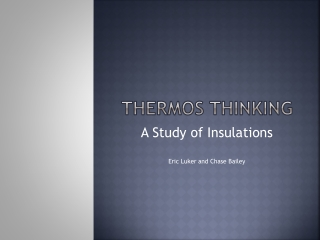Download PresentationThermos Thinking

# Thermos Thinking

Télécharger la présentation## Thermos Thinking

- - - - - - - - - - - - - - - - - - - - - - - - - - - E N D - - - - - - - - - - - - - - - - - - - - - - - - - - -
##### Presentation Transcript

1. Thermos Thinking A Study of Insulations Eric Luker and Chase Bailey

2. History • The thermos, originally called the vacuum flask was invented by Sir James Dewar in 1892. • It was constructed of two flasks, one sitting inside the other and only touching at the neck, where they were joined together. • The air was partially removed from the gap between the flasks leaving a vacuum in the space.

3. The question What is the difference in heat flux between vacuum, air, and foam insulated thermos?

4. The Setup Dimensions • The thermos is a cube with 30 centimeter wall. • The inner and outer wall thicknesses are 2 millimeters. • The space between the inner and outer walls is 1 centimeter. Assumptions • Constant temperature inside and outside the thermos • Constant heat flux To simplify the calculations we chose the following dimensions and made the following assumptions:

5. Theoretical Thermos

6. Analysis Using these equations we calculated the resistances of the resistance circuit. Since we were comparing different insulations we calculated the resistance for each one. To make sure that we had appropriately guessed the temperatures of the walls we iterated the process. We did this by checking the temperatures relative to the constant flux.

7. Results

8. Conclusion • Thermoses/Coolers work better keeping their contents cold than they do keeping their contents hot. This is because often times the difference between atmospheric temperature and cold temperature is much less than atmospheric and hot. • Polyurethane Foam(PUR) is the best insulator of the three types under analysis. • Air is the worst insulator under the given conditions

9. Things to Note • Thickness of the insulation affects the resistance of the PUR foam and the air but not the radiation in the Vacuum • An average value for the coefficient of conduction was used for PUR instead of the values relating to the different temperature conditions. This was due to a the information available. • We expected the radiation to have the greatest resistance but this was not the case. Looking over our calculations, we determined they were accurate. There could perhaps be some effects that we are not taking into account.

10. Appendices Excel Worksheet used for repetitive calculations

11. Sample of hand calculations performed